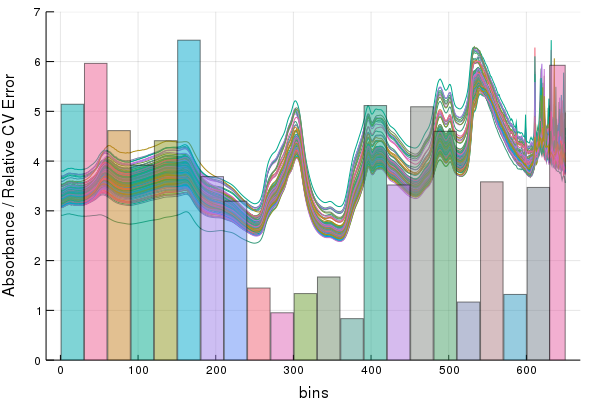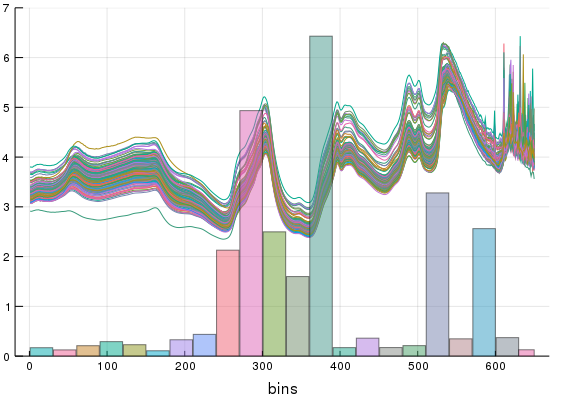SIPLS

# Stacked Interval Partial Least Squares

Here's a post I kind of debated making... I once read a paper stating that SIPLS was "too complicated" to implement, and used that as an argument to favor other methods. SIPLS is actually pretty simple, highly effective, and it has statistical guarantees. What's complicated about SIPLS is providing it to end-users without shielding them from the internals, or leaving them with a pile of hard to read low level code. I decided, the way to go for 'advanced' methods, is to just provide convenience functions. Make life easier for an end-user that knows what they are doing. Demo's are for helping ferry people along and showing at least one way to do things, but there's no golden ticket one-line generic code-base here. Providing it, would be a mistake to people who would actually rely on using this sort of method.

### 4-Steps to SIPLS

1. Break the spectra's columnspace into invervals (the size can be CV'd but below I just picked one), then we CV PLS models inside each interval.
2. On a hold out set(or via pooling), we find the prediction error of our intervals
3. Those errors are then reciprocally weighted
4. Apply those weights to future predictions via multiplication and sum the result of each interval model.

### 1. Crossvalidate the interval models

``````MaxLvs = 10
CVModels = []
CVErr = []
Intervals = MakeIntervals( size(calib1), 30 );
for interval in Intervals
IntervalError = repeat([0.0], MaxLvs);
Models = []

for Lv in MaxLvs:-1:1
for ( i, ( Fold, HoldOut ) ) in enumerate(KFoldsValidation(10, calib1, caliby))
if Lv == MaxLvs
KFoldModel = PartialLeastSquares(Fold[:,interval], Fold; Factors = Lv)
push!( Models, KFoldModel )
end

Predictions = Models[i]( HoldOut[:, interval]; Factors = Lv)
IntervalError[Lv] += SSE( Predictions, HoldOut)
end
end
OptimalLv = argmin(IntervalError)
push!(CVModels, PartialLeastSquares(calib1[:, interval], caliby; Factors = OptimalLv) )
push!(CVErr,    IntervalError[OptimalLv] )
end``````

For fun, we can view the weights of each intervals relative error on the CV'd spectra with this lovely convenience function,

``IntervalOverlay(calib1, Intervals, CVErr)``### 2. Validate

``````VErr = []
IntervalError = repeat([0.0], MaxLvs);
for (model, interval) in enumerate(Intervals)
push!(VErr, SSE( CVModels[model](valid1[:,interval]), validy) )
end``````

### 3. Make reciprocal weights

``StackedWeights = stackedweights(VErr);``

We can recycle that same plot recipe to observe what this weighting function does for us. After calling the stacked weights function we can see how much each interval will contribute to our additve model. In essence, the weights make the intervals with lower error contribute more to the final stacked model,### 4. Pool predictions on test set and weight results

``````Results = zeros(size(tst1));
for (model, interval) in enumerate(Intervals)
Results += CVModels[model](tst1[:,interval]) .* StackedWeights[model]
end

RMSE( Results, tsty)``````

`> 4.09`

The RMSE from the SIPLS model is ~0.6 units less then that which we can observe from the same dataset using base PLSR in my Calibration Transfer Demo. This is actually really fast to run too. Every line in this script (aside from importing CSV) runs in roughly ~1-2 seconds.